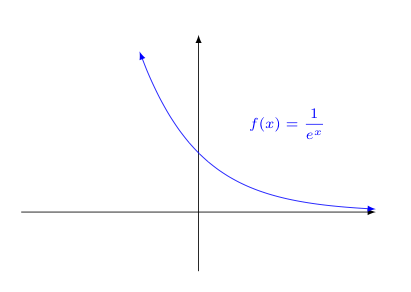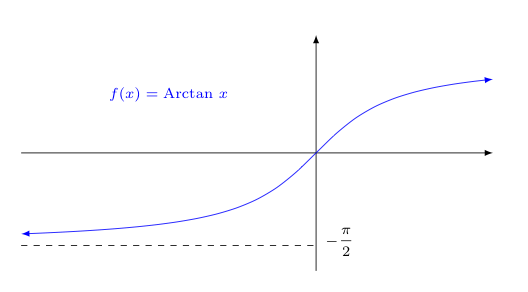## Limits at Infinity

Consider the graph of the function $\displaystyle{f(x)=\frac{1}{e^x}}$ shown below.Clearly, looking at the graph, the height of this function appears to get closer and closer to a height of zero, as we look at $x$ values farther and farther to the right.

That is to say, it appears that $f(x) \rightarrow 0$ as $x \rightarrow +\infty$.

It seems like we should be able to use the language of limits to describe this behavior -- after all, when we say $f(x) \rightarrow L$ as $x \rightarrow c$, we equivalently write $$\lim_{x \rightarrow c} f(x) = L$$ In other functions, such as $f(x) = \textrm{Arctan } x$ shown below, if we look at the behavior of the graph as our $x$ values move farther and farther left (i.e., as $x \rightarrow -\infty$) we sometimes see the height of the graph getting closer and closer to some other value.

In the graph below, it appears that as $f(x) \rightarrow \pi/2$ as $x \rightarrow -\infty$.Notice in both of the examples above, graphically we are seeing a horizontal asymptote at the limiting height.

So the question is, can we extend the idea of limits to include not only the limiting value of a function as $x$ approaches some finite value $c$, but also the limiting value of a function as $x$ approaches infinity (or negative infinity)?

Recall that we say $\lim_{x \rightarrow c} f(x) = L$ when we mean that we can keep $f(x)$ arbitrarily close to $L$ by keeping $x$ sufficiently close to $c$ (with the possible exception of what happens when $x=c$).

So how would this play out when $c$ is replaced by $\infty$? What would we mean by "$x$ being sufficiently close to $\infty$"?

As we have mentioned before, infinity is not a number, so we can't insist that we keep $x$ within $\delta$ units of it, like we do in the normal $\epsilon-\delta$ definition of a limit, but...

Recall how we do have an intuitive feeling for one number being "closer" to infinity than another. Remember the fourth grader, who when asked the question "Which number is closer to infinity, 13 or 20 billion?'', responds with 20 billion because it is way larger than 13.

Let's use that idea here too. Let us replace the phrase "keeping $x$ sufficiently close to $\infty$" with "keeping $x$ sufficiently large".

Now, with this in mind, how do we design a definition for this new notion of a limit at infinity that is analogous to the $\epsilon-\delta$ definition of a limit at some $x=c$? Let us remind ourselves of the latter...

 $\epsilon-\delta$ Definition for the limit of $f(x)$ as $x$ approaches $c$ $\lim_{x \rightarrow c} f(x)=L$ means that for any $\epsilon>0$, we can find a $\delta>0$ such that if $0<|x-c|<\delta$, then $|f(x)-L| < \epsilon$.

So if one wishes to keep $f(x)$ within $\epsilon$ units of $L$ (for any $\epsilon > 0$), one can accomplish this by "keeping $x$ sufficiently close to $c$". Equivalently, there is some $\delta > 0$ such that keeping $0 \lt |x-c| \lt \delta$ will accomplish this.

Notice, we can now say something similar for a "limit at infinity"...

If one wishes to keep $f(x)$ within $\epsilon$ units of $L$ (for any $\epsilon > 0$), one should "keep $x$ sufficiently large". Equivalently, there is some number $N$ such that keeping $x > N$ will accomplish this.

Substituting this language in the above definition, we can formally define what we mean by a limit at infinity:

 Definition for the limit of $f(x)$ as $x$ approaches $\infty$ $\lim_{x \rightarrow \infty} f(x)=L$ means that for any $\epsilon>0$, we can find some number $N$ such that if $x > N$, then $|f(x)-L| < \epsilon$.

It remains to consider limits at negative infinity -- but this is dealt with in an extremely similar and natural way...

 Definition for the limit of $f(x)$ as $x$ approaches $-\infty$ $\lim_{x \rightarrow \infty} f(x)=L$ means that for any $\epsilon>0$, we can find some number $N$ such that if $x < N$, then $|f(x)-L| < \epsilon$.